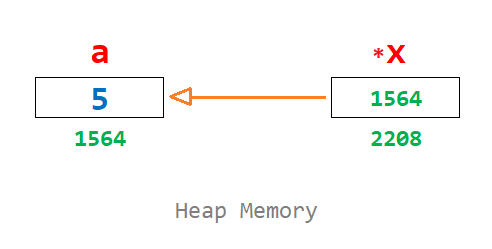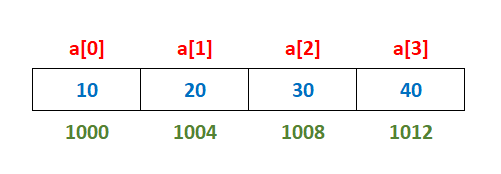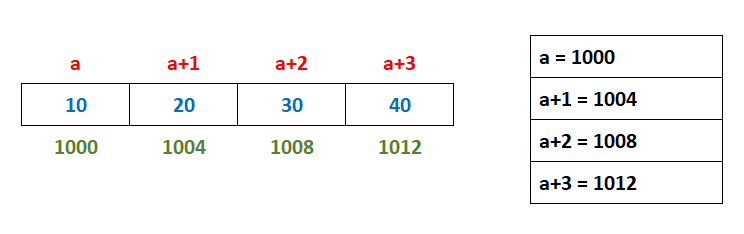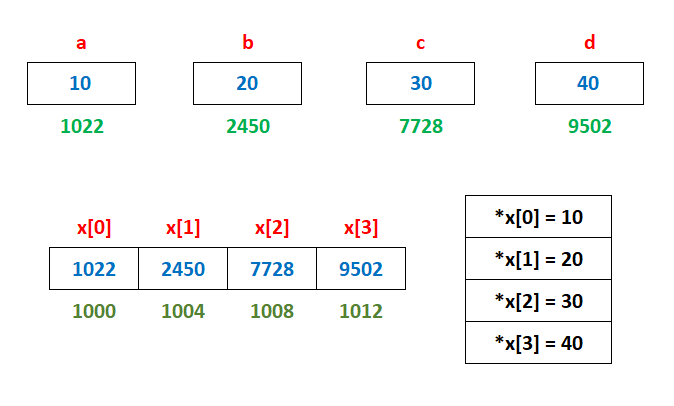# Pointer in C Programming

###### Pointer and Structure in C

In this lesson, we will understand what is Pointer in C Programming along with some examples.

## What is Pointer in C

A Pointer is a variable that stores or points to the memory address of another variable.

In C programming, when we declare a variable, it allocates some space in the heap memory. Each allocated space has a unique memory address which is used by the compiler to access the values stored in them. With the help of pointers, we can directly work with the memory address of variables.### Syntax of Declaring a Pointer Variable in C

``datatype *variable_name;``

#### Example

``````int *a       // An integer type pointer variable
float *b     // A float type pointer variable
double *c    // A double type pointer variable``````

## Store the Memory Address of a Variable in Pointer

To store the memory address of a variable in a pointer. We have to use the & operator. The & operator represents the address of a variable.

#### Example

``````int a=5     // An integer type variable
int *x      // An integer type pointer variable
x=&a        // Store the memory address of variable a to pointer variable x using & operator``````

The image given below shows how the pointer works in computer memory.In the above image, the integer variable a and the pointer variable *x allocates a memory space having address 1564 and 2208 respectively.

The & operator is used to store the address of variable a in pointer variable *x using the statement x=&a. So we can say that the variable x points to the value of the variable a by storing its address.

Note: The above memory addresses are imaginary address used for explaining the pointer. The actual address varies from computer to computer.

## Access the Value of a Variable using Pointer

To access the value of a variable using a pointer, use the * operator with the pointer variable. See the examples given below.

#### Example 1

``````#include <stdio.h>
#include <conio.h>

int main()
{
int a=5, *x;
x=&a;

printf("Value of a = %d\n",a);
printf("x points to address = %u\n",x);
printf("x points to value = %d\n",*x);
return 0;
}``````

#### Output

```Value of a = 5
x points to address = 6356748
x points to value = 5```

Note: The memory address in the above output may vary from computer to computer.

#### Example 2

C program to input principal, rate, time and calculate the simple interest using pointer variables. The Formula of calculation simple interest is (principal*rate*time)/100.

``````#include <stdio.h>
#include <conio.h>

int main()
{
int p,r,t,si,*pr,*rt,*tm,*sint;
pr=&p;
rt=&r;
tm=&t;
sint=&si;

printf("Enter Principal: ");
scanf("%d",pr);
printf("Enter Rate: ");
scanf("%d",rt);
printf("Enter Time: ");
scanf("%d",tm);

*sint=(*pr * *rt * *tm)/100;
printf("Simple Interest = %d",*sint);
return 0;
}``````

#### Output

```Enter Principal: 5000
Enter Rate: 10
Enter Time: 1
Simple Interest = 500```

In the above program, we have used pointer variables to solve the problem of simple interest. The variables p, r and t are used to allocate integer type memory whose address are stored in the pointer variables *pr, *rt and *tm.

We have stored the values from the user input in the variables p, r and t using pointer variables pr, rt and tm.

The simple interest is calculated and stored in the variable sint by accessing the values pointed by the variables pr, rt and tm using * operator.

Note: Use * operator to change, store or access the value of a pointer variable.

#### Example 3

C program to print all the even numbers between 1 to 10 using pointer variable.

``````#include <stdio.h>
#include <conio.h>

int main()
{
int i,*x;
x=&i;

for(*x=2; *x<=10; *x=*x+2)
{
printf("%d ",*x);
}
return 0;
}``````

#### Output

`2 4 6 8 10`

#### Example 4

C program to input a number and check if is a prime number or not using pointer variables. A prime number is a number which is divisible by 1 and itself.

``````#include <stdio.h>
#include <conio.h>

int main()
{
int i,n,c,*x,*nm,*fc;
x=&i;
nm=&n;
fc=&c;
*fc=0;

printf("Enter a number ");
scanf("%d",nm);

for(*x=1; *x<=*nm; *x=*x+1)
{
if(*nm % *x==0)
{
*fc=*fc+1;		// counting the factors
}
}

if(*fc==2)
{
printf("Prime Number");
}
else
{
printf("Not Prime Number");
}
return 0;
}``````

#### Output

```Enter a number 13
Prime Number```

#### Example 5

C program to input a number and find the sum of even digits using pointer variables.

``````#include <stdio.h>
#include <conio.h>

int main()
{
int i,n,s,d,*x,*nm,*sum,*dg;
x=&i;
nm=&n;
sum=&s;
dg=&d;
*sum=0;

printf("Enter a number ");
scanf("%d",nm);

while(*nm>0)
{
*dg=*nm%10;
if(*dg % 2==0)
{
*sum=*sum+*dg;
}
*nm=*nm/10;
}

printf("Sum of even digits = %d",*sum);
return 0;
}``````

#### Output

```Enter a number 54768
Sum of even digits = 18```

## Pointer to Pointer

We can also store the address of a pointer variable to another pointer variable. It is known as Pointer to Pointer. A Pointer to Pointer variable is also called Pointer's Pointer. It is declared with two **

#### Example

C program to show the use of pointer to pointer variable.

``````#include <stdio.h>
#include <conio.h>

int main()
{
int a=5,*b,**c;

b=&a;      // store the address of variable a
c=&b;      // store the address of variable b

printf("Value of a = %d\n",a);

printf("b points to address = %u\n",b);
printf("b points to value = %d\n",*b);

printf("c points to address = %u\n",c);
printf("c indirectly points to value %d",**c);
return 0;
}``````

#### Output

```Value of a = 5
b points to address = 6356748
b points to value = 5
c points to address = 6356744
c indirectly points to value 5```

Note: The memory address in the above output may vary from computer to computer.

## Pointer and Array

We know that an array is a collection of similar data type element. The elements in an array are stored at a contiguous memory location. The base address of an array is the address of the 0th element of the array. The name of the array also gives the base address of the array.

#### Example

``int a[]= {10,20,30,40};``In the above image, the base address of the array is 1000. As int data type allocate 4 bytes in memory, so the address of the next element in the array will be at a difference of 4 bytes as shown above. Remember that the above-given addresses are imaginary they may vary from computer to computer.

We can store the base address of an array in a pointer and thus use the pointer to access the rest of the address in the array by simply incrementing the pointer by 1 each time. See the example given below.#### Example

C program to show the use of array pointer.

``````#include <stdio.h>
#include <conio.h>

int main()
{
int a[]= {10,20,30,40};
int i,*p;
p=a;	// store the base address of the array to pointer p

for(i=0; i<4; i++)
{
printf("Address of a[%d] = %u and Value = %d\n",i,(p+i),*(p+i));
}
return 0;
}``````

#### Output

```Address of a = 6356728 and Value = 10
Address of a = 6356732 and Value = 20
Address of a = 6356736 and Value = 30
Address of a = 6356740 and Value = 40```

## Array of Pointers

We can declare an array of pointers where each element of the array is a pointer and can store the memory address of multiple variables of the same data type.#### Example

C program to show the use of pointers' array.

``````#include <stdio.h>
#include <conio.h>

int main()
{
int *x;
int a=10,b=20,c=30,d=40,i;

x=&a;
x=&b;
x=&c;
x=&d;

for(i=0; i<4; i++)
{
printf("Value of x[%d] = %u and Points to = %d\n",i,x[i],*x[i]);
}
return 0;
}``````

#### Output

```Value of x = 6356728 and Points to = 10
Value of x = 6356724 and Points to = 20
Value of x = 6356720 and Points to = 30
Value of x = 6356716 and Points to = 40```